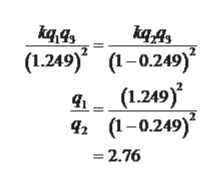Three particles are fixed on an x axis. Particle 1 of charge q1 is at x = -a and particle 2 of charge q2 is at x = +a. If their net electrostatic force on particle 3 of charge Q is to be zero, what must be the ratio q1/q2 when particle 3 is at (a) x = +0.249a and (b) x = +2.28a?

Question

Three particles are fixed on an x axis. Particle 1 of charge q1 is at x = -a and particle 2 of charge q2 is at x = +a. If their net electrostatic force on particle 3 of charge Q is to be zero, what must be the ratio q1/q2 when particle 3 is at (a) x = +0.249a and (b) x = +2.28a?

Step 1

a)

The required ratio of t...help_outlineImage TranscriptioncloseК44, а4 (1.249) 1-0.249) (1.249) 42 (-0.249) 2.76 fullscreen

Want to see the full answer?

See Solution

Want to see this answer and more?

Our solutions are written by experts, many with advanced degrees, and available 24/7

See Solution
Tagged in

Electric Charges and Fields